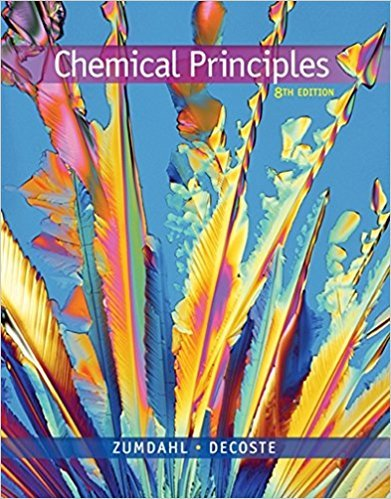×
Get Full Access to Chemical Principles - 8 Edition - Chapter 10 - Problem 10.91
Get Full Access to Chemical Principles - 8 Edition - Chapter 10 - Problem 10.91

×

# Consider the relationship ln1K2 5 2DH RT 1 DS R TheISBN: 9781305581982 176

## Solution for problem 10.91 Chapter 10

Chemical Principles | 8th Edition

• Textbook Solutions
• 2901 Step-by-step solutions solved by professors and subject experts
• Get 24/7 help from StudySoup virtual teaching assistantsChemical Principles | 8th Edition

4 5 1 399 Reviews
29
1
Problem 10.91

Consider the relationship ln1K2 5 2DH RT 1 DS R The equilibrium constant for some hypothetical process was determined as a function of temperature (in kelvins) with the results plotted below. 1000 T(K) ln ( K) 10. 1.0 2.0 3.0 20. 30. 40. From the plot, determine the values of DH8 and DS8 for this process. What would be the major difference in the ln(K) versus 1/T plot for an endothermic process as compared to an exothermic process?

Step-by-Step Solution:
Step 1 of 3

Ligand Binding: Oxygen Binding to Hemoglobin & Myoglobin • Hemoglobin and myoglobin are part of the same superfamily-­‐ they have the same 3D structure but they do not have the same amino acid sequence (primary structure) • Hemoglobin has four subunits and myoglobin only have one-­‐ this gives them different properties Ligand binding is extremely specific • A ligand is a molecule (smaller than a protein) that bind

Step 2 of 3

Step 3 of 3

##### ISBN: 9781305581982

Since the solution to 10.91 from 10 chapter was answered, more than 386 students have viewed the full step-by-step answer. The full step-by-step solution to problem: 10.91 from chapter: 10 was answered by , our top Chemistry solution expert on 12/23/17, 04:53PM. The answer to “Consider the relationship ln1K2 5 2DH RT 1 DS R The equilibrium constant for some hypothetical process was determined as a function of temperature (in kelvins) with the results plotted below. 1000 T(K) ln ( K) 10. 1.0 2.0 3.0 20. 30. 40. From the plot, determine the values of DH8 and DS8 for this process. What would be the major difference in the ln(K) versus 1/T plot for an endothermic process as compared to an exothermic process?” is broken down into a number of easy to follow steps, and 78 words. Chemical Principles was written by and is associated to the ISBN: 9781305581982. This full solution covers the following key subjects: . This expansive textbook survival guide covers 20 chapters, and 2754 solutions. This textbook survival guide was created for the textbook: Chemical Principles, edition: 8.

Unlock Textbook Solution